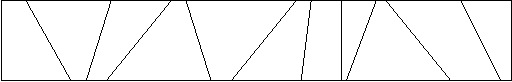# 题目

## Description

Calculate the number of toys that land in each bin of a partitioned toy box.
Mom and dad have a problem - their child John never puts his toys away when he is finished playing with them. They gave John a rectangular box to put his toys in, but John is rebellious and obeys his parents by simply throwing his toys into the box. All the toys get mixed up, and it is impossible for John to find his favorite toys.

John’s parents came up with the following idea. They put cardboard partitions into the box. Even if John keeps throwing his toys into the box, at least toys that get thrown into different bins stay separated. The following diagram shows a top view of an example toy box.For this problem, you are asked to determine how many toys fall into each partition as John throws them into the toy box.

## Input

The input file contains one or more problems. The first line of a problem consists of six integers, $n$ $m$ $x_1$ $y_1$ $x_2$ $y_2$ . The number of cardboard partitions is $n$ $(0 < n \leq 5000)$ and the number of toys is $m$ $(0 < m \leq 5000)$ . The coordinates of the upper-left corner and the lower-right corner of the box are $(x_1,y_1)$ and $(x_2,y_2)$ , respectively. The following $n$ lines contain two integers per line, $U_i$ $L_i$ , indicating that the ends of the i-th cardboard partition is at the coordinates $(U_i,y_1)$ and $(L_i,y_2)$ . You may assume that the cardboard partitions do not intersect each other and that they are specified in sorted order from left to right. The next $m$ lines contain two integers per line, $X_j$ $Y_j$ specifying where the j-th toy has landed in the box. The order of the toy locations is random. You may assume that no toy will land exactly on a cardboard partition or outside the boundary of the box. The input is terminated by a line consisting of a single $0$ .

## Output

The output for each problem will be one line for each separate bin in the toy box. For each bin, print its bin number, followed by a colon and one space, followed by the number of toys thrown into that bin. Bins are numbered from $0$ (the leftmost bin) to $n$ (the rightmost bin). Separate the output of different problems by a single blank line.

## Hint

As the example illustrates, toys that fall on the boundary of the box are “in” the box.

# 代码

-------------本文结束感谢您的阅读-------------